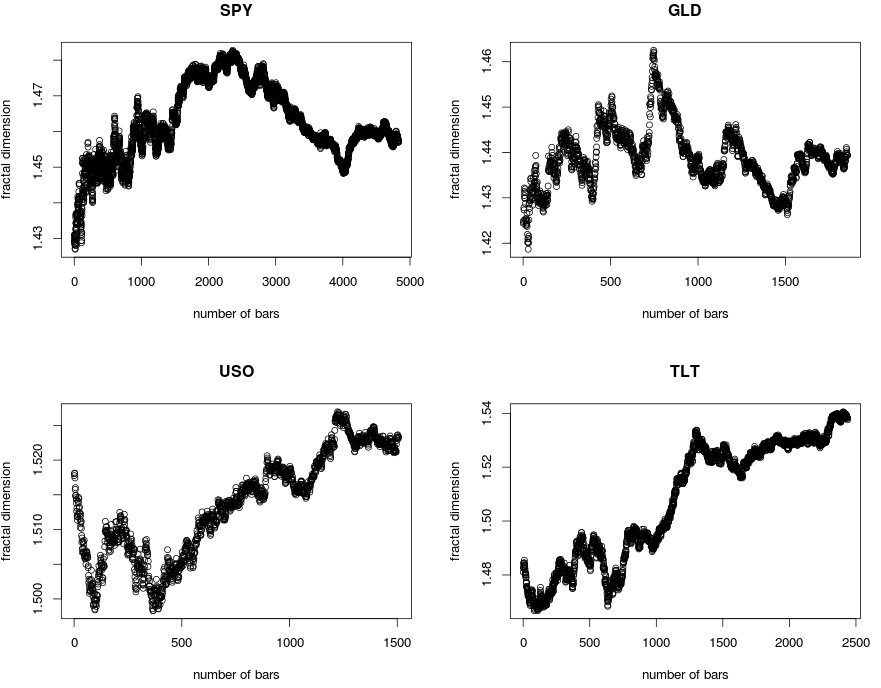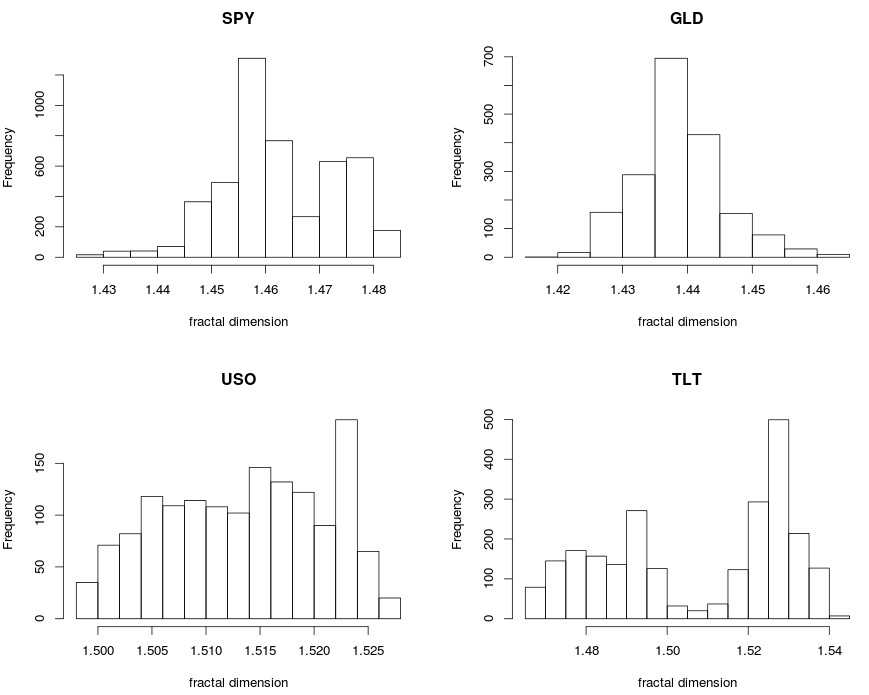Using R in trading: Is the fractal dimension constant in financial timeseries?

Recently I wrote several posts on the making of predictions using Chaos in financial time series. These posts were inspired by a Nature article on the prediction of chaotic series and the apparently wonderful results obtained by the paper’s authors. Today I want to write a post about the fundamental assumption within that paper, which is that the chaos related properties of a financial time series remain constant through time. This is the core assumption made within the paper and evaluating it is fundamental to understanding whether the methodologies proposed within the paper can work or not. On today’s article I will show you some R code to calculate the value of the fractal dimension as a function of time and we’ll discuss the results obtained for different financial time series.

If financial time series are chaotic and the underlying factors that determine the series don’t change then we should expect their chaos related properties to converge to some underlying real value for the series as a function of time. The R code above calculates the fractal dimension as a function of time from a 1000 bar start point till the end of the financial time series for a chosen financial instrument’s daily data downloaded from Yahoo (using the QuantMod package). This allows us to see whether the fractal dimension of the series remains constant or starts to converge to some value or whether the fractal dimension simply changes substantially and seemingly erratically through time. After performing this observation we can judge whether a Chaos based prediction mechanism makes sense – if betting that the next variation will cause the least perturbation in the time series’ fractal dimension makes sense – or whether the symbol does not conform to this assumption and henceforth a chaos based prediction will be futile.

The graph below shows the evolution of the fractal dimension as a function of the number of bars after the first 1000 bars for four different ETF instruments. The variation in most cases is rather small – with standard deviations in the 0.01-0.02 range – suggesting that in most cases the fractal dimension does change slowly as a function of time. It is worth pointing out that for USO and TLT the variations are particularly close to the 1.5 mark which is the value we would expect for a random walk. A series with a fractal dimension of 1.5 – or rather close to it – should be efficient meaning that making a profit from it should not be possible despite our best efforts. If a series has a fractal dimension of 1.5 then the uncertainty in short term predictions should be large enough that making a profit from judging directionality should simply not be possible. Even if the series has a chaos value that never changes if that value is at 1.5 you are most likely out of luck.With this in mind we need to be wary of both the fractal dimension value and its standard deviation. The standard deviation in the fractal dimension for the USO series is 0.007 but it’s mean is 1.513 while the series with the highest deviation is the SPY series with a deviation of 0.02 and a mean of 1.462. The USO series might conform best to the assumption that the fractal dimension remains constant but the predictions might be the least useful while in the case of the SPY the series conforms less to the assumption but is more inefficient. The best series for prediction from the four above might in fact be GLD where the standard deviation is the lowest at 0.0065 and the mean is the most distant from 1.5 at 1.4388. This is probably why when I have looked into series predictions GLD has often yielded the best results while series like USO generally yield very inaccurate predictions.

The distributions of the fractal dimension show us some interesting information as well. The GLD and SPY distributions are rather well defined while the distribution for USO is quite broad and the TLT case shows two apparent distributions, one centered around 1.49 and another centered closed to 1.53.You can see on the TLT graph that the values for the fractal dimension were initially showing variations around 1.49 and then the value for the fractal dimension shot up to around 1.53, where it is right now. This strong move in the fractal dimension may obey to some fundamental changes in the actual factors that determined the value of TLT. It is not surprising to see that this change in the fractal dimension in TLT happens right through the financial crisis (2008-2009).As a matter of fact when looking deeper into when the gradient of the fractal dimension is the strongest I find that this happens specifically during times of turmoil. This completely negates the usefulness of this prediction method because it shows that it is most likely to fail when we need it the most. If variations in the fractal dimension are bound to happen when we need them not to – if we’re making predictions based on a constant fractal dimension – then if changes in the fractal dimension happen when the market goes into crisis it means that we are bound to get the most errors around times where we would desire to get the least. Of course the effect might be small enough to allow for useful prediction in a series like GLD – were overall variations are small – while in others like TLT the effect is so big that the prediction mechanism might be useless.

Despite this it is really interesting to note how the behavior of some financial series has changed tremendously while that of other series has remained rather constant. I definitely wonder if the value of the fractal dimension and its gradient at any given point in time – plus the relationship between the fractal dimension of the different series – might indeed be a leading indicator for market changes. If you would like to learn more about time series analysis and how you too can create automated tradingplease consider joining Asirikuy.com, a website filled with educational videos, trading systems, development and a sound, honest and transparent approach towards automated trading.strategies